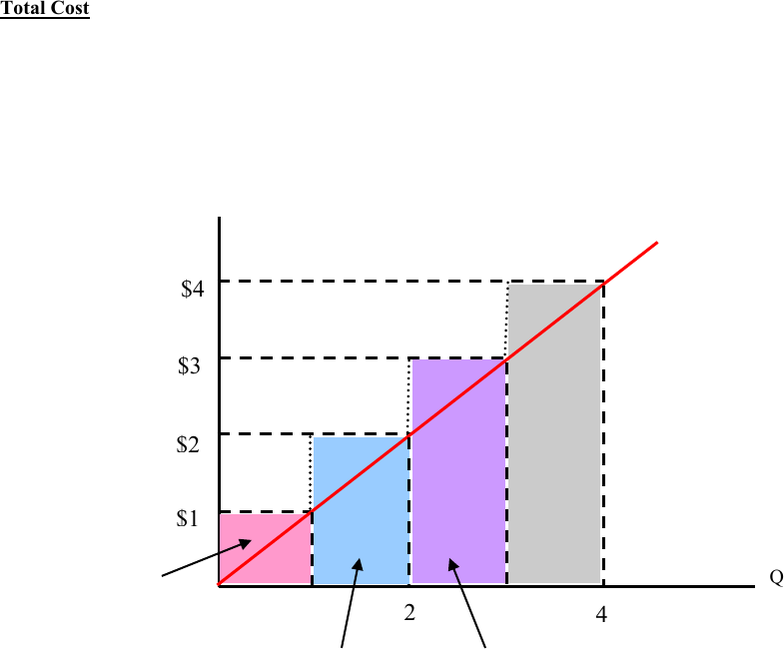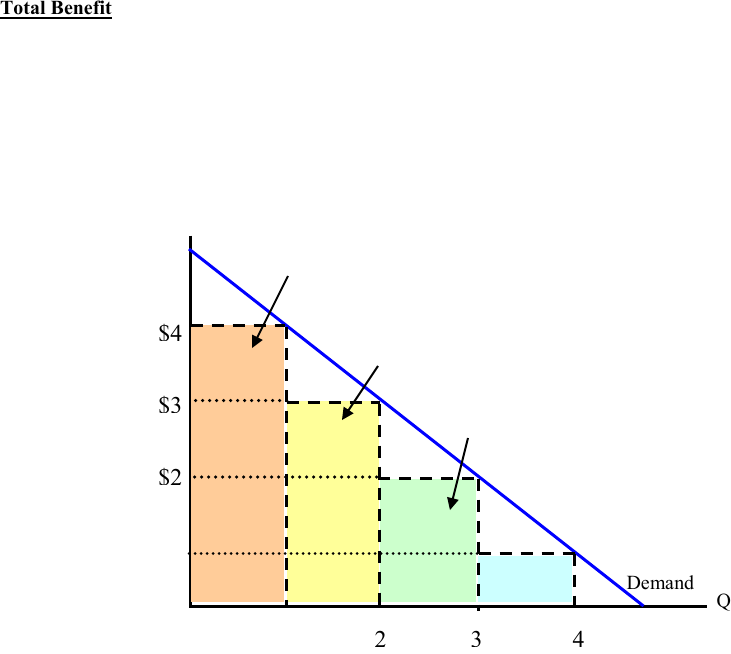# ECON 131 Lecture Notes - Lecture 3: Marginal Cost, Marginal Utility, Economic Equilibrium

14 views5 pages5.a. Total Cost and Total Benefit
Total Cost
It is the cost of producing a certain number of units of a good.
Graphically, it can be expressed as the area underneath the supply curve up to the quantity produced. See
Figure 5.a.1 below.
Figure 5.a.1: The total cost to produce the first unit of the good is \$1 (Area 1). The cost of
producing an additional unit of the good is an additional \$2 (Area 2) for a total cost of \$3 (Area 1
+Area 2) to produce a total of 2 units. If the producer wanted to producer a third unit, the
additional cost of producing that extra unit would be \$3 (Area 3). The total cost of producing 3
units of the good would be Area 1 + Area 2 + Area 3 or \$1 + \$2 + \$3 = \$6.
NOTE: The amount that it costs to produce one more unit of a good is known as marginal cost
(each individual area). Hence:
Total Cost = Sum of Marginal Costs.
1
2
3
4
\$1
\$2
\$3
\$4
P
Supply
Area 1
Area 2
Area 3
Unlock document

This preview shows pages 1-2 of the document.
Unlock all 5 pages and 3 million more documents.Total Benefit
It is the maximum amount a consumer would be willing to pay for a certain number of units of a good. It
can also be thought of as the total benefit a consumer gets for a certain amount of a good or the total value
that they place upon that amount.
Graphically, it can be expressed as the area underneath the consumer’s demand curve up to the quantity
purchased. See Figure 5.a.2 below.
Figure 5.a.2: To attain one unit of a good, a consumer is willing to pay \$4 (Area 1). For an
additional unit the consumer would be willing to pay an additional \$3 for that second unit (Area
2) for a total of \$7 for the two units (Area 1 + Area 2). For the next additional unit, the consumer
would only be willing to pay \$2 in order to obtain that additional third unit (Area 3). Therefore,
the total benefit this consumer receives from consuming 3 units of the good is Area 1 + Area 2 +
Area 3 or \$4 + \$3 + \$2 = \$9.
NOTE: The amount that the consumer is willing to pay in order to obtain one more unit is known
as marginal benefit (each individual area). Hence:
Total Benefit = Sum of Marginal Benefits.
1
2
3
4
\$1
\$2
\$3
\$4
Q
P
Demand
Area 1
Area 2
Area 3
Unlock document

This preview shows pages 1-2 of the document.
Unlock all 5 pages and 3 million more documents.

## Document Summary

It is the cost of producing a certain number of units of a good. Graphically, it can be expressed as the area underneath the supply curve up to the quantity produced. Figure 5. a. 1: the total cost to produce the first unit of the good is (area 1). The cost of producing an additional unit of the good is an additional (area 2) for a total cost of (area 1. +area 2) to produce a total of 2 units. If the producer wanted to producer a third unit, the additional cost of producing that extra unit would be (area 3). The total cost of producing 3 units of the good would be area 1 + area 2 + area 3 or + + = . Note: the amount that it costs to produce one more unit of a good is known as marginal cost (each individual area).